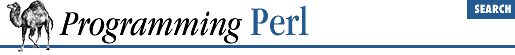home | O'Reilly's CD bookshelfs | FreeBSD | Linux | Cisco | Cisco Exam

#### 7.2.13 English - Use English or awk Names for Punctuation Variables

```use English;
...
if (\$ERRNO =~ /denied/) { ... }```

This module provides aliases for the built-in "punctuation" variables. Variables with side effects that get triggered merely by accessing them (like \$0 ) will still have the same effects under the aliases.

For those variables that have an awk (1) version, both long and short English alternatives are provided. For example, the ``` \$/``` variable can be referred to either as ``` \$RS``` or as ``` \$INPUT_RECORD_SEPARATOR``` if you are using the English module.

Here is the list of variables along with their English alternatives:

Perl English Perl English
@_ ``` @ARG``` \$? ``` \$CHILD_ERROR```
\$_ ``` \$ARG``` \$! ``` \$OS_ERROR```
\$& ``` \$MATCH``` \$! ``` \$ERRNO```
\$ ``` ```` ``` \$PREMATCH``` \$@ ``` \$EVAL_ERROR```
\$ ``` '``` ``` \$POSTMATCH``` \$\$ ``` \$PROCESS_ID```
\$+ ``` \$LAST_PAREN_MATCH``` \$\$ ``` \$PID```
\$. ``` \$INPUT_LINE_NUMBER``` \$< ``` \$REAL_USER_ID```
\$. ``` \$NR``` \$< ``` \$UID```
\$/ ``` \$INPUT_RECORD_SEPARATOR``` \$> ``` \$EFFECTIVE_USER_ID```
\$/ ``` \$RS``` \$> ``` \$EUID```
\$| ``` \$OUTPUT_AUTOFLUSH``` \$( ``` \$REAL_GROUP_ID```
\$, ``` \$OUTPUT_FIELD_SEPARATOR``` \$( ``` \$GID```
\$, ``` \$OFS``` \$) ``` \$EFFECTIVE_GROUP_ID```
\$\ ``` \$OUTPUT_RECORD_SEPARATOR``` \$) ``` \$EGID```
\$\ ``` \$ORS``` \$0 ``` \$PROGRAM_NAME```
\$" ``` \$LIST_SEPARATOR``` \$] ``` \$PERL_VERSION```
\$; ``` \$SUBSCRIPT_SEPARATOR``` \$^A ``` \$ACCUMULATOR```
\$; ``` \$SUBSEP``` \$^D ``` \$DEBUGGING```
\$% ``` \$FORMAT_PAGE_NUMBER``` \$^F ``` \$SYSTEM_FD_MAX```
\$= ``` \$FORMAT_LINES_PER_PAGE``` \$^I ``` \$INPLACE_EDIT```
\$- ``` \$FORMAT_LINES_LEFT``` \$^P ``` \$PERLDB```
\$~ ``` \$FORMAT_NAME``` \$^T ``` \$BASETIME```
\$^ ``` \$FORMAT_TOP_NAME``` \$^W ``` \$WARNING```
\$: ``` \$FORMAT_LINE_BREAK_CHARACTERS``` \$^X ``` \$EXECUTABLE_NAME```
\$^L ``` \$FORMAT_LINEFEED``` \$^O ``` \$OSNAME```7.2.12 DynaLoader - Automatic Dynamic Loading of Perl Modules7.2.14 Env - Import Environment Variables